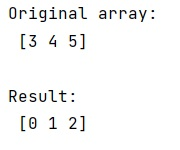# Are numpy arrays passed by reference?

Learn, how to zip two 1d numpy array to 2d numpy array in Python?
Submitted by Pranit Sharma, on January 20, 2023

NumPy is an abbreviated form of Numerical Python. It is used for different types of scientific operations in python. Numpy is a vast library in python which is used for almost every kind of scientific or mathematical operation. It is itself an array which is a collection of various methods and functions for processing the arrays.

## Passing numpy arrays by reference

Basically, in python, all the variable names are passed by reference. Suppose that we are passing an array into a function where we are subtracting some values from the original array.

When Python evaluates an assignment, the right-hand side is evaluated before the left-hand side. Any operation on an array creates a new array; it does not modify the arr in-place.

Any operation makes the local variable a reference to the new array. It does not modify the value originally referenced by arr which was passed to function.

Let us understand with the help of an example,

## Python code to pass numpy arrays by reference

```# Import numpy
import numpy as np

# Creating a numpy array
arr = np.array([3, 4, 5])

# Display original array
print("Original array:\n",arr,"\n")

# Defining a function
def fun(a):
a = a - 3
return a

# Calling the function
res = fun(arr)

# Display the result
print("Result:\n",res)
```

Output:What's New (MCQs)

Top Interview Coding Problems/Challenges!

IncludeHelp's Blogs

Languages: » C » C++ » C++ STL » Java » Data Structure » C#.Net » Android » Kotlin » SQL
Web Technologies: » PHP » Python » JavaScript » CSS » Ajax » Node.js » Web programming/HTML
Solved programs: » C » C++ » DS » Java » C#
Aptitude que. & ans.: » C » C++ » Java » DBMS
Interview que. & ans.: » C » Embedded C » Java » SEO » HR
CS Subjects: » CS Basics » O.S. » Networks » DBMS » Embedded Systems » Cloud Computing
» Machine learning » CS Organizations » Linux » DOS
More: » Articles » Puzzles » News/Updates